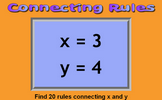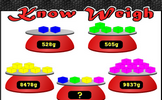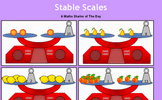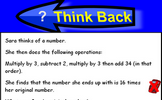# Algebra

### Term 2 starting in week 5 :: Estimated time: 2 weeks

• Find a rule one step
• Find a rule - two step
• Forming expressions
• Substitution
• Formulae
• Forming equations
• Solve simple one-step equations
• Solve two step equations
• Find pairs of values
• Enumerate possibilities

This page should remember your ticks from one visit to the next for a period of time. It does this by using Local Storage so the information is saved only on the computer you are working on right now.

## Lesson Starters

Here are some suggestions for whole-class, projectable resources which can be used at the beginnings of each lesson in this block.

### 1st Lesson#### Connecting Rules

Give 20 rules connecting x and y given their values.

### 2nd Lesson### 3rd Lesson#### Stable Scales

Solve these balance puzzles by taking the same away from both sides. An introduction to linear equations.

### 4th Lesson#### Lost Sheep

Which algebraic expression is the odd one out?

### 5th Lesson#### Think Back

A problem which can be answered by forming an algebraic equation then solving it.

### 6th Lesson#### THOAN

THOAN stands for 'Think of a number' and there are four randomly generated THOAN puzzles to solve.

Some of the Starters above are to reinforce concepts learnt, others are to introduce new ideas while others are on unrelated topics designed for retrieval practice or and opportunity to develop problem-solving skills.

White Rose ResourcesEnd of block assessments provide a quick progress check at the end of each block of learning to make sure students have understood the content covered. This Scheme of Learning was produced by White Rose Maths and is used here with permission granted on 30th June 2021.For All: